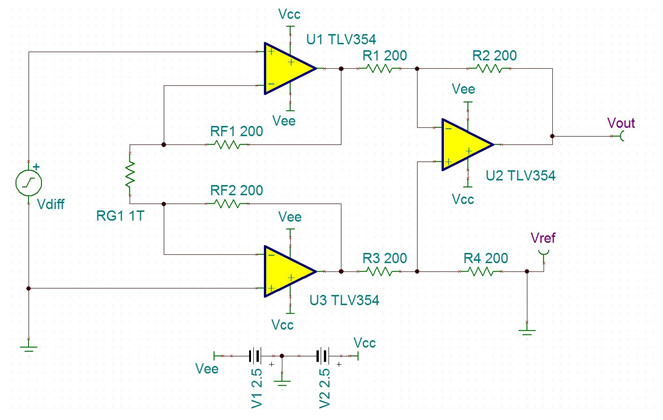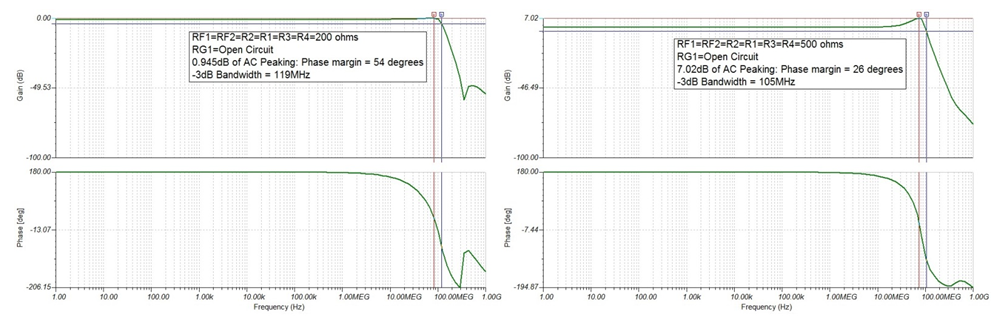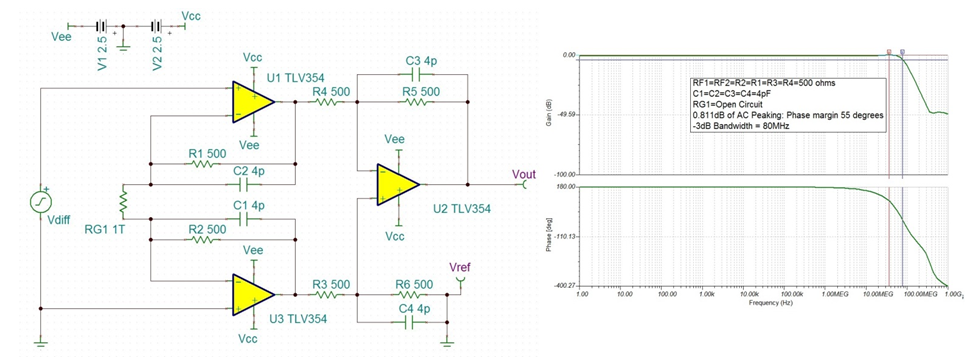Other Parts Discussed in Post: TINA-TI

In this post, I’ll show how to design a cost-optimized, discrete, wide-bandwidth instrumentation amplifier using the TLV3544. Instrumentation amplifiers are used for their high input impedance and ability to convert differential voltages to single-ended voltages. Fast current sensing, precision data acquisition, vibration analysis, microphone pre-amplification, ADC drivers and medical instrumentation are all applications that need instrumentation amplifiers with wide bandwidth.

TLV354x devices provide a rail-to-rail input/output, 200MHz unity gain bandwidth (GBW) and 150V/µs slew rate, which is designed for the applications I just mentioned. Figure 1 shows the standard three-operational amplifier (op amp) topology.Figure 1: Discrete three-op-amp topology using the TLV3544

The input stage uses dual noninverting amplifiers that enable high impedance at both inputs, whose gain is defined by RF1 = RF2 and RG1. The output stage consists of a difference amplifier with a low impedance output, whose gain is set by R2 = R4 and R1 = R3. The reference voltage, input stage gain and output stage gain define the output voltage, shown in Equation 1:Note that the tolerance of the resistors in the instrumentation amplifier will negatively affect the CMRR and gain error of the circuit. That is why there is a cost and performance trade-off between discrete and integrated instrumentation amplifiers.

The bandwidth of instrumentation amplifiers is bounded by three characteristics: the open-loop gain (Aol) of the op amp, the noise gain (Gn) and filtering. Both Aol and Gn are covered in the TI Precision Labs training series on bandwidth (see part 3, “TI Precision Labs – Op Amps: Bandwidth 3” – viewing requires a myTI login). The output-stage difference amplifier’s noise gain determines the circuit’s bandwidth.

The TLV3544, like many high-speed amplifiers, will have stability issues if the feedback resistors are too large. Figure 2 simulates the effects of 200Ω feedback resistors versus 500Ω feedback resistors. Note that 45 degrees of phase margin (PM) is necessary for stable operation. Increasing the resistor values allows for lower power consumption and larger RG1 values to set the gain.Figure 2: TINA-TI™ software frequency response showing AC peaking, PM and bandwidth with 200Ω (54 degrees PM) versus 500Ω (26 degrees PM) feedback resistors

Since the instrumentation amplifier is discrete, you have access to the feedback paths, which allows you to compensate for AC peaking. By placing capacitors in the feedback path, you introduce multiple poles that create a low-pass filter and attenuate the peaking. Equation 2 calculates the -3dB frequency (fp) of this low-pass filter:Figure 3 shows the schematic and frequency response. Remember that adding a filter will affect the overall bandwidth of the circuit.Figure 3: TINA-TI software schematic and frequency response of compensated feedback paths

Since this instrumentation amplifier topology only requires three op amps, the fourth op amp provided by the TLV3544 can serve as a reference buffer for single-supply systems or as an integrator for high-pass filtering of the input.

Thus, you can use the TLV3544 to make a discrete wide-bandwidth instrumentation amplifier that is ideal for cost-optimized, high-precision, wide-bandwidth applications.

• I've answered the recommendations in an email.

However, I find that it is important to state that you can remove RG1 and simulate the Wide BW INA in a gain of 1V/V. By removing RG1, RG1 in Equation 1 effectively approaches infinity. This approximately reduces the gain term to (1 + 0)(R2/R1).

I often simulate gain=1V/V using RG1 = 1T ohm so I do not have to delete the resistor, as shown in the schematics above.

-Cole

• Dear

I have one 0-50mV signal and the frequency range is between 10KHz to 5MHz. I want to measure the signal very accurately. (the price is not important)

I have simulated your circuit. But this is not perfect for my application.

Do you have any recommendations for me?

Can I open RG1 to have unity gain?

it's my email: golhaaa@gmail.com

Regards